4.2.6 CDM Models, halo structure and rotation of spirals

CDM models predict a halo structure which is responsible for the rotation curve of the spiral galaxies. Halo structures and rotation curves are therefore closely connected problems. Let us assume that halos are spherical.

Gunn and Gott (1972) concluded that the gravitational collapse could lead to the formation of virialized halos with almost isothermal profiles. A tempting assumption for the halo density distribution is therefore the so called non-singular "isothermal" sphere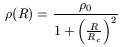(71)

defined with two parameters: the central density,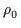, and the core radius, Rc. In this isothermal profile (d/dR)(R = 0) = 0 and(R = 0) =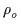is finite, two desirable properties for a density profile. Out to RRc, the density remains more or less flat, i.e., there is a "core" of radius Rc. Note that, in this case, the "circular velocity", V, is defined as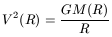(72)

where M(R) is the mass in a sphere of radius R. It can then be deduced that the circular velocity is given by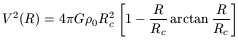(73)

which is an increasing function of R, asymptotically reaching Vmax = V(R =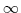) given by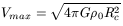(74)

which might be an undesirable property (an asymptotically Keplerian curve would be preferable).

Other types of halos have been reviewed by Bertschinger (1998) and others. Recently Navarro, Frenk and White (1996, 1997) deduced from their CDM models that halos should be described by the so called "universal" or NFW profiles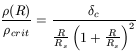(75)

where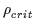is the density of the critical Einstein-de Sitter Universe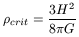(76)

and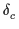, the characteristic contrast density (dimensionless) and Rs, the scale radius, are the parameters of the profile. It is singular,(R = 0) =, which is certainly an "unpleasant" property, even if the mass M(0) converges. The NFW profile was called "universal" because the authors found it in a large variety of halo masses, spanning 4 orders of magnitude, from individual galaxies to cluster halos, as well as for a large variety of cosmological scenarios. Some authors (e.g. Avila-Reese, Firmani and Hernandez, 1998) deduced that the density profile depends on the environment, with the NFW appropriate only for isolated halos. The circular velocity of this NFW halo can be calculated by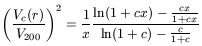(77)

where V200 is the circular velocity at R200, called the "Virial" radius. This virial radius is that radius for which <> = 200, where <> is the mean density in a sphere of radius R200. Cole and Lacey (1996) showed that this radius approximately separates the virialized and infall regions. The parameter c, called the concentration, is defined as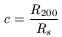(78)

and is dimensionless. x is simply R/R200.

As V2002 = GM200/R200 = G(200 × 3H2/8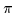G)(4(R200)3/3)/R200 = 100H02R2002, we have V200 = 10H0R200, or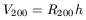(79)

if we measure V200 in kms-1 and R200 in kpc. Also: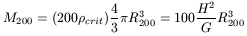(80)

therefore M200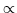R2003V2003. If the luminous mass were proportional to the halo mass, M200, and if Vopt were related to V200 (Vopt is the disk velocity at Ropt, the optical radius), then a relation similar to the observational Tully-Fisher relation would be obtained. In conventional astronomical units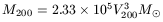(81)

where V200 is to be expressed in km/s. The Tully-Fisher relation clearly establishes a relation between the luminosity and some power of the optical rotational velocity, LVopt3 or LVopt4. (The exponent depends on the wavelength of the observations. The higher value of 4 is for the infrared. See van den Bosch (1999) for a recent critical review).

The obtention of the Tully-Fisher relation from the outcoming halo density distribution presents some problems. The slope obtained and the scattering of the points agree with the observations, but the theoretical curve is displaced with the observational curve (Frenk et al. 1997). Or equivalently, it is possible to vary the free parameters to match the Tully-Fisher relation but then the amplitude of the galaxy luminosity function is not matched. This is at present a failure of theoretical models. The number of predicted dark halos is excessive. Navarro, Frenk and White (1996) suggested several possibilities: other cosmological parameters, the existence of a large number of halos with no visible component, the non-detection of many existent low surface brightness galaxies, etc.

Even if the observational Tully-Fisher relation is basically reproduced, there is still no convincing explanation for the numerical outputs. As Navarro (1998) remarks: "Our analysis has made use of this surprisingly tight relation between disk luminosity and rotation speed but provides no firm clues to elucidate its origin".

There is a general argument based on Shu (1982) that does not explain the Tully-Fisher relation but does introduce some light. If the total luminosity of a galaxy is roughly LRopt2 (whereis the central surface brightness, notably the same for all spirals, and Ropt the optical radius) and if the total mass within the optical radius is roughly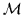RoptVopt2 (where Vopt is the asymptotic observed velocity) and if/L is roughly a constant, then RoptVopt2/. Ifis really a constant RoptVopt2 and therefore LVopt4. This argument relies on the constancy of, which so far has no explanation.

The NFW circular velocity reaches a maximum at R2Rs = 2R200/c and declines beyond that radius. NFW density profiles are two-parametric and it is possible to choose V200 and c to characterize the halos, or the equivalent set of characteristic density and halo mass. A very exciting result of these theoretical models is that the two free parameters show a clear correlation. The reason behind this is that the halo density reflects, and is proportional to, the true density when the halo was formed, with the initial small halos being denser because they formed earlier, when the density of the expanding Universe was higher. But we also know that, due to the hierarchical halo formation, more massive halos were born later. Then, the existence of decreasing functions(t) and M200(t) implies a correlation between(t) and M200(t). Therefore, in practice, rotation curves are intrinsically one-parametric. Figure 18 plots the circular velocity curves for different values of the concentration, c. Low values of the concentration parameters denote slowly rising curves, i.e. small ancient galaxies.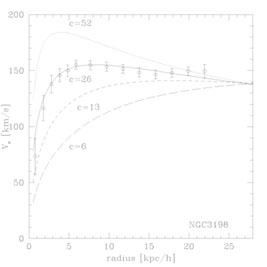Figure 18. NFW circular velocity profiles for different values of the concentration. The circular velocity at the virial radius, V200, is chosen to match the rotation speed in the outskirts of the galaxy, and varies from 100 to 130 km s-1 from top to bottom. As illustrated in this figure for NGC 3198, most galaxy rotation curves can be adequately parametrized. The best fit value of the c parameter ( cobs26 in this case) provides a quantitative measure of the shape of the rotation curve. Low values of cobs denote slowly rising curves, while high values of cobs indicate steeply rising, flat or declining rotation curves. Furthermore, cobs constitutes a firm upper limit to the concentration of the halo, since the fit neglects the contribution of the luminous component. From Navarro (1998).

We saw above that the "maximum disk" hypothesis provides halo circular velocities. Do NFW halo profiles fit these profiles? Are disks really maxima? Do isothermal profiles provide a better fit than NFW profiles? A comparison of the theoretically predicted halos and the observations is necessary to answer these questions and to test the models. This comparison was made by Navarro (1998), who adopted about 100 disk galaxies from published observations and tried to deduce the NFW free parameter in each case. This analysis was also made under the isothermal halo assumption.

For this task, we should not forget that galaxies also have visible components, i.e. that spirals possess a disk and a bulge. For the surface brightness of the disk, Navarro (1998) assumed, as usual, an exponential disk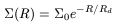(82)

where=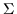(R = 0) and Rd, the disk radial scale length, are two parameters. Exponential optical disks constitute a reasonable zeroth-order description, but there is perhaps a misuse in the literature, as above discussed. It is however an appropriate and almost a necessary assumption in studies similar to that of Navarro.

For the surface brightness of the bulge it is assumed that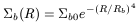(83)

where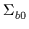and Rb are two parameters. Navarro assumed the same M/L ratio in the bulge and in the disk, and taht this ratio was another free parameter.

It is also usually assumed that the halo responds "adiabatically" to the growth of the disk (Barnes and White, 1984; Flores et al. 1993), which was also assumed by Navarro, Frenk and White (1996, 1997). This means a variation of the NFW profile within the disk region. Certainly, the formation of the disk must somewhat modify the halo density profile, probably in a way that is very difficult to model. This assumption was introduced in order to avoid disagreements with observations of the rotation of dwarf galaxies and therefore, as a correction of an initial theoretical failure. In fact, the rotation curve of dwarf galaxies indicates that the halo circular velocity rises almost linearly, which would mean a constant density (i.e. a halo core) in clear contradiction with the NFW profiles. As mentioned above, this hypothesis of the adiabatic response of the halo to the formation of the disk also alleviates the problem of the halo-disk conspiracy.

The results indicate that theoretical models must introduce a higher degree of sophistication, because even isothermal profiles give similar or better results, specially for low surface brightness galaxies. Moreover, observational rotation curves are very often well fitted by halos with no disk and no bulge! This type of fitting is meaningless, but Cosmology could benefit from it. The concentration parameter, c, obtained after this peculiar halo-only fitting provides an upper limit which can be compared with the theoretical predictions on c. The standard CDM model in general predicts higher concentrations than the upper limits obtained. Therefore, if the theoretical models are considered an efficient basis to interpret the observations, then cosmological models with small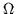(0.3) and large(0.7) are favoured.

A puzzling observation within the DM interpretation of rotation curves is the absence of correlation between the asymptotic velocity of disks and the orbital velocity in binary systems. If the asymptotic velocity, Vrot, is found in a region dynamically dominated by the halo, and the orbital velocity, Vorb, of a galaxy considered the secondary would, clearly, reflect the total mass of the primary, Vrot and Vorb should correlate. Navarro (1998) seeks the explanation in his Fig. 19 (top-right: logV200 versus logVrot). If we observe the solid line in this figure, we see that disks with Vrot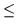150kms-1 have V200 > Vrot and disks with Vrot > 150kms-1 are all predicted to have similar halo velocities, V200200kms-1. Then, disk-dominated galaxies would be surrounded by halos of approximately the same mass.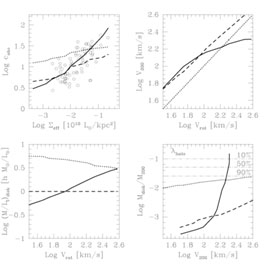Figure 19. Top-left: Rotation curve shape characterized by the parameter cobs, the value of the concentration deduced by the observations of the rotation curve, versus effective surface brightness (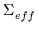= L/R2disk). Top-right: V200 versus Vrot, commented in text. In all four plots: dotted lines assume that Vrot = V200 and only (M/L)disk is adjusted to match the Tully-Fisher relation. Short-dashed lines assume that (M/L)disk = hM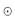/Lin all galaxies; only V200 is varied to match the Tully-Fisher relation. Solid lines correspond to varying both (M/L)disk and V200 so as to match the Tully-Fisher relation and the- cobs correlation. From Navarro (1999).

This explanation of rotation curves remains incomplete. Samples do not contain dwarf galaxies. The Navarro sample is probably also lacking early bulge-rich galaxies. In Fig. 26 there are two other curves, the M/L =h curve and the varying M/L curve, which are not unreasonable (for instance, Vrot = V200 implies very small variations of M/L) but which, however, do not imply the same conclusion. Even if the solid line shows us a change in slope, the increasing function does not firmly predict the complete absence of correlation. The discussion of Navarro concerning this point is very illustrative but the puzzling behaviour of binary galaxies is not completely cleared up. The existence of an upper limit of the halo mass needs further justification.

Furthermore, Navarro and Steimetz (1999) find it difficult to reconcile the theory with data for the Milky Way and with the Tully-Fisher relation; they consider that substantial revision of the theoretical models is needed.

As a general conclusion, observational rotation curves are not incompatible with NFW halos, but the confrontation seems somewhat discouraging. We have examined the work of Navarro (1998) in some detail because it is probably the most serious and complete study linking the observed properties and the model outputs for rotation curves. However, either the observations do not constitute a proof of the CDM models, or dynamic ingredients other than halo and disk density profiles are necessary to study the rotation of spirals. Considering the success of the models in explaining and predicting other observational facts, we would suggest this second possibility as more plausible. In particular, we will later argue that ignoring magnetic fields in the interpretation of the rotation curves could be unrealistic.